Tags Math

In this post I want to show some interesting definitions and theorems about equivalence relations & classes, and groups.

Relations are an important topic in algebra. Conceptually, a relation is a statement aRb about two elements of a set. If the elements are integers, then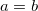is a relation, and so is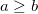.

Here's a formal set-theoretic definition:

(I) Binary relation: A binary relation on a set A is a collection of ordered pairs of elements of A. In other words, it is the subset of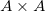. More generally, a binary relation between two sets A and B is a subset of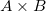.

Note it says ordered pairs. What this means is that the order of elements in a relation is important. Intuitively, given the relation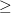and the set of integers, it's clear thatdoes not generally imply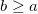.

An important sub-class of relations we'll be most interested with is the equivalence relations:

(II) Equivalence relation: A relationon a set is called an equivalence relation if it's reflexive, symmetric and transitive:

• Reflexive: for all a in S it holds that.
• Symmetric: for all a and b in S it holds that if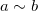then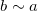.
• Transitive: for all a, b and c in S it holds that ifand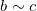then.

Examples: equality is an equivalence relation, but greater-or-equal is not, asdoesn't imply- the symmetric condition doesn't hold.

Another important equivalence relation is the congruence modulo an integer,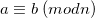.

(III) Example: Let G be a group and H a subgroup of G. For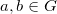, defineif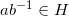. Thenis an equivalence relation on G.

Proof: To prove that some relation is an equivalence relation, we have to prove the three properties of equivalence relations.

Reflexive: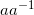is the identity element e. However, since H is a subgroup of G, it means that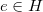. Therefore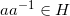, so.

Symmetric: Assume that. Since H is a subgroup, then this element has an inverse in H: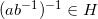. Using the associative law of groups several times it's possible to show that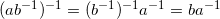So,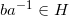, hence.

Transitive: Givenand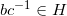.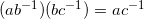So,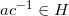, hence.

Thus, we've proved that thisis an equivalence relation. Let's see a concrete application of the result we've just proved:

Consider that if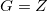with the operation +, and H is the subgroup consisting of all multiples of some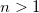, thenactually means that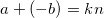for some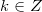. In other words. This proves that congruence modulo n is an equivalence relation, since it's a special case of (III).

(IV) Equivalence class: Ifis an equivalence relation on S, then [a], the equivalence class of a is defined by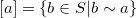For example, let's take the integers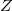and define an equivalence relation "congruent modulo 5". For instance,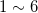. The congruence class of 1 modulo 5 (denoted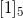) is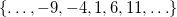.

(V) Group partition: Ifis an equivalence relation on S, then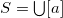for all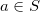, and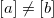implies that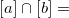. In other words,partitions S into disjoint equivalence classes.

Proof: the first part is easy. Since always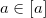, then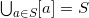. To prove the second part, we'll show that if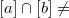then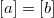.

Suppose that, and let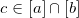. Thereforeand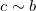. Butis an equivalence relation and thus is transitive and symmetric. So. But this means that a and b are in the same equivalence class:. Q.E.D.